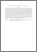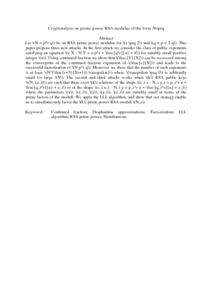# Cryptanalysis on prime power RSA modulus of the form N=prq

## Citation

Kamel Ariffin, Muhammad Rezal and Shehu, Sadiq (2016) Cryptanalysis on prime power RSA modulus of the form N=prq. International Journal of Applied Mathematical Research, 5 (4). pp. 167-175. ISSN 2227-4324

## Abstract

Let $$N = p^r q$$ be an RSA prime power modulus for $$r \geq 2$$ and $$q < p < 2 q$$. This paper propose three new attacks. In the first attack we consider the class of public exponents satisfying an equation $$e X - N Y = u p^r + \frac{q^r}{u} + Z$$ for suitably small positive integer $$u$$. Using continued fraction we show that $$\frac{Y}{X}$$ can be recovered among the convergents of the continued fraction expansion of $$\frac{e}{N}$$ and leads to the successful factorization of $$N p^r q$$. Moreover we show that the number of such exponents is at least $$N^{\frac{r+3}{2(r+1)}-\varepsilon}$$ where $$\varepsilon \geq 0$$ is arbitrarily small for large $$N$$. The second and third attacks works when $$k$$ RSA public keys $$(N_i,e_i)$$ are such that there exist $$k$$ relations of the shape $$e_i x - N_i y_i = p_i^r u + \frac{q_i^r}{u} + z_i$$ or of the shape $$e_i x_i - N_i y = p_i^r u + \frac{q_i^r}{u} + z_i$$ where the parameters $$x$$, $$x_i$$, $$y$$, $$y_i$$, $$z_i$$ are suitably small in terms of the prime factors of the moduli. We apply the LLL algorithm, and show that our strategy enable us to simultaneously factor the $$k$$ prime power RSA moduli $$N_i$$.Preview
PDF (Abstract)
Cryptanalysis on prime power RSA modulus of the form N=prq.pdfView Item